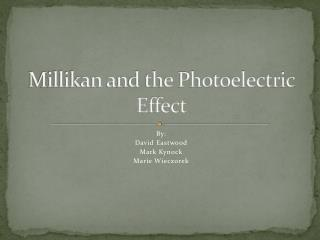DownloadDownload PresentationMillikan and the Photoelectric Effect

# Millikan and the Photoelectric Effect

Télécharger la présentation## Millikan and the Photoelectric Effect

- - - - - - - - - - - - - - - - - - - - - - - - - - - E N D - - - - - - - - - - - - - - - - - - - - - - - - - - -
##### Presentation Transcript

1. Millikan and the Photoelectric Effect By: David Eastwood Mark Kynock Marie Wieczorek

2. Robert Andrews Millikan • Born: March 22, 1868 in Morison, Illinois. • Died: December 19, 1953 in San Marino, California. • Second son of Reverend Silas Franklin Millikan and Mary Jane Andrews. • His favourite undergraduate subjects were Greek and Math. • Obtained a Masters in Physics. • Was appointed Fellow in Physics at Columbia University. • Got his PhD. In 1895 for “research on the polarization of light emitted by incandescent surfaces - using for this purpose molten gold and silver at the U.S. Mint.” • He has been awarded the Comstock Prize of the National Academy of Sciences, the Edison Medal of the American Institute of Electrical Engineers, the Hughes Medal of the Royal Society of Great Britain, and the Nobel Prize for Physics 1923. He was also made Commander of the Legion of Honour, and received the Chinese Order of Jade.

3. Robert Millikan • By 1916 Robert Millikan found that the magnitude of an electrons charge was 1.6 x 10-19 C. With this, Millikan set out to prove that Einstein’s thoughts on quantum nature of light were wrong. • Millikan made photoelectric tubes with emitters made of many metals. He measured the stopping potential for different frequencies, for each metal that he used. Using his experimental charge of an electron, he calculated the values for the maximum kinetic energy for every frequency using E=qV

4. In Millikan’s case E was the max kinetic energy of the potoelectrons q was the charge on an electrons Which made the equation Ek(max)= eVstop Millikan then graphed his results

5. To relate the graphs of Ek(max) versus f to Einstein’s, he solved for Ek(max) Ek(max)= hf- W Notice how the equation relates to the equation of a straight line y=mx+b Comparing the two equations, Planks constant, h, is the slope, m, and the Work function, W, is the y intercept, b.

6. The Electron volt. • eV – electron volt is needed when using the photoelectric formula. The electron volt is the energy gained by one electron as it falls through the potential difference of one volt and it is used because the Joules that are used in the photoelectric effect are very small therefore the eV was developed. • E=qV • 1eV=(1e)(1V) • 1eV=(1.60x10 • 1eV= 1.60x10 -19 C)(1V) J -19

7. Equations and Sample Problem E=qV V= f hf0=W or f0=W/h Table 18.1 on p.853 • The stopping potential for electrons emitted from the surface of lead is 3.57V. What is the frequency of the photons that are striking the metal surface?

8. Problems. • 1. The frequency of the photons is 1.1 x 10^15 Hz. What is the most likely type of surface that the photons are striking?

9. 2. A metal has 1.258 x 10^-13 C and a stopping potential of 0.547V. How many electron volts are there in this metal?

10. 3. If the stopping potential is 5.68 x 10^6V and the frequency is 8.62 x 10^12 Hz, what is the wavelength?

11. Bibliography • http://www.google.ca/imgres?imgurl=http://reich-chemistry.wikispaces.com/file/view/millikan.jpeg/31451689/millikan.jpeg&imgrefurl=http://reich-chemistry.wikispaces.com/Slaney.Camara.atomictimeline&usg=__Yws8Cwa_-gSi7CAxxH-mLDGsQIg=&h=300&w=248&sz=46&hl=en&start=0&zoom=1&tbnid=549r1z0iu-ALmM:&tbnh=147&tbnw=140&ei=5Yi9TbPJFaLz0gH9iYCwBg&prev=/search%3Fq%3Drobert%2Bmillikan%26hl%3Den%26sa%3DX%26biw%3D1419%26bih%3D715%26tbm%3Disch%26prmd%3Divnso&itbs=1&iact=rc&dur=156&page=1&ndsp=36&ved=1t:429,r:1,s:0&tx=63&ty=104 • http://nobelprize.org/nobel_prizes/physics/laureates/1923/millikan-bio.html • http://www.google.ca/imgres?imgurl=http://www.capitolpride.org/images/symbol_lambda.png&imgrefurl=http://www.capitolpride.org/glbt_symbols.shtml&usg=__0TlmncHjJOfQoutNvuJdQzOCVoQ=&h=400&w=240&sz=8&hl=en&start=0&zoom=1&tbnid=xcvfiUyRoPn3KM:&tbnh=143&tbnw=86&ei=AJK9TY_xOuia0QG42u2yBg&prev=/search%3Fq%3Dwavelength%2Bsymbol%26um%3D1%26hl%3Den%26sa%3DN%26biw%3D1436%26bih%3D715%26tbm%3Disch&um=1&itbs=1&iact=hc&vpx=123&vpy=32&dur=772&hovh=290&hovw=174&tx=68&ty=191&page=1&ndsp=28&ved=1t:429,r:0,s:0 • 2003 McGraw-Hill Ryerson Physics Textbook • 1989 Cutnell-Johnson Second Edition Physics Textbook Physics Fourth Edition, D.C Heath and Company, Lexington, Massachusetts, Toronto